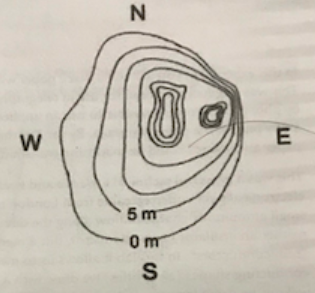# Problem: The diagram represents a contour map of hilly island. Copy it into your lab notebook. The outer contour of the figure is at sea level. All points on any one particular contour line are at same elevation (vertical distance above sea-level). The distance between any two contour lines in the diagram represents the horizontal distance between points on the two different countours.  North is up. The same diagram could also represent the contours of the electrical potential of two positively charged objects with irregular shapes (for example charges stored on a rubber sheet). Assume that the outer part of the figure is at zero potential. Each contour line going towards the center represents an equipotential surface with a potential 5 V greater than the previous contour. a) Where is the electric field particularly strong? Show at least three places. Explain your reasoning with reference to Q1(a).b) There are two areas of high potential on the diagram. What is the value (in Volts) of c)  each of these high potential peaks? c) What path would require you to use the least amount of force to push a test charge from zero potential to the point of highest potential of one of the charged objects? (Choose the same one you labeled B in 1b) above.) Explain your reasoning. d) Would you actually do less work to move the test charge from 0 V to the voltage at B by using the path selected in 2c), or would the actual path make no difference?

###### FREE Expert Solution

(a)

Electric field:

$\overline{){\mathbf{E}}{\mathbf{=}}{\mathbf{-}}\frac{\mathbf{∆}\mathbf{V}}{\mathbf{∆}\mathbf{x}}}$

ΔV = -E•Δx

The electric field is very high when a small change, Δx, produces a large change in potential.

93% (243 ratings)###### Problem Details

The diagram represents a contour map of hilly island. Copy it into your lab notebook. The outer contour of the figure is at sea level. All points on any one particular contour line are at same elevation (vertical distance above sea-level). The distance between any two contour lines in the diagram represents the horizontal distance between points on the two different countours.  North is up.The same diagram could also represent the contours of the electrical potential of two positively charged objects with irregular shapes (for example charges stored on a rubber sheet). Assume that the outer part of the figure is at zero potential. Each contour line going towards the center represents an equipotential surface with a potential 5 V greater than the previous contour.

a) Where is the electric field particularly strong? Show at least three places. Explain your reasoning with reference to Q1(a).

b) There are two areas of high potential on the diagram. What is the value (in Volts) of c)  each of these high potential peaks?

c) What path would require you to use the least amount of force to push a test charge from zero potential to the point of highest potential of one of the charged objects? (Choose the same one you labeled B in 1b) above.) Explain your reasoning.

d) Would you actually do less work to move the test charge from 0 V to the voltage at B by using the path selected in 2c), or would the actual path make no difference?